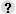• Matrices
• Algebra
• Geometry
• Graphs and functions
• Trigonometry
• Coordinate geometry
• Combinatorics
 Suma y resta Producto por escalar Producto Inversa
 Monomials Polynomials Special products Equations Quadratic equations Radical expressions Systems of equations Sequences and series Inner product Exponential equations Matrices Determinants Inverse of a matrix Logarithmic equations Systems of 3 variables equations
 2-D Shapes Areas Pythagorean Theorem Distances
 Graphs Definition of slope Positive or negative slope Determine slope of a line Equation of a line Equation of a line (from graph) Quadratic function Parallel, coincident and intersecting lines Asymptotes Limits Distances Continuity and discontinuities
 Sine Cosine Tangent Cosecant Secant Cotangent Trigonometric identities Law of cosines Law of sines
 Equations of a straight lineParallel, coincident and intersecting lines Distances Angles in space Inner product

 Compare ratios: word problems When Peter works out, he spends 12 minutes doing cardio and 18 minutes doing resistance training. Rogert's workout consists of 19 minutes of cardio and 17 minutes of resistance training. Who does a lower ratio of cardio to resistance training? In Peter's workout routine, the ratio of cardio to resistance training is 12 to 18. In Rogert's, the ratio is 19 to 17. We want to figure out which ratio is higher: and We can compare the ratios more easily if we express them as percentages. First write the ratio as a decimal. Then convert the decimal to a percentage. Do the same thing for Now compare the percentages. 66.667%111.765% 66.667% is smaller than 111.765%. Peter had a lower ratio of cardio to resistance training. Which is the best buy: $4.08 for a 16-ounce box of cereal or$4.50 for an 18-ounce box of cereal? The ratio of the first buy is 4.08 to 16. The ratio of the second buy is 4.50 to 18. We want to figure out which ratio is lower: and We can compare the ratios more easily if we express them as percentages. First write the ratio as a decimal. Then convert the decimal to a percentage. Do the same thing for Now compare the percentages. 25.5%25% 25% is smaller than 25.5%. The better buy is the second one.